# sfepy.solvers.ts_solvers module¶

Time stepping solvers.

class sfepy.solvers.ts_solvers.AdaptiveTimeSteppingSolver(conf, nls=None, context=None, **kwargs)[source]

Implicit time stepping solver with an adaptive time step.

Either the built-in or user supplied function can be used to adapt the time step.

Kind: ‘ts.adaptive’

For common configuration parameters, see Solver.

Specific configuration parameters:

Parameters
t0float (default: 0.0)

The initial time.

t1float (default: 1.0)

The final time.

dtfloat

The time step. Used if n_step is not given.

n_stepint (default: 10)

The number of time steps. Has precedence over dt.

quasistaticbool (default: False)

If True, assume a quasistatic time-stepping. Then the non-linear solver is invoked also for the initial time.

adapt_funcallable(ts, status, adt, context, verbose)

If given, use this function to set the time step in ts. The function return value is a bool - if True, the adaptivity loop should stop. The other parameters below are collected in adt, status is the nonlinear solver status, context is a user-defined context and verbose is a verbosity flag. Solvers created by Problem use the Problem instance as the context.

dt_red_factorfloat (default: 0.2)

The time step reduction factor.

dt_red_maxfloat (default: 0.001)

The maximum time step reduction factor.

dt_inc_factorfloat (default: 1.25)

The time step increase factor.

dt_inc_on_iterint (default: 4)

Increase the time step if the nonlinear solver converged in less than this amount of iterations for dt_inc_wait consecutive time steps.

dt_inc_waitint (default: 5)

The number of consecutive time steps, see dt_inc_on_iter.

name = 'ts.adaptive'
output_step_info(ts)[source]
solve_step(ts, nls, vec, prestep_fun)[source]

Solve a single time step.

class sfepy.solvers.ts_solvers.BatheTS(conf, nls=None, context=None, **kwargs)[source]

Solve elastodynamics problems by the Bathe method.

The method was introduced in .

 Klaus-Juergen Bathe, Conserving energy and momentum in nonlinear dynamics: A simple implicit time integration scheme, Computers & Structures, Volume 85, Issues 7-8, 2007, Pages 437-445, ISSN 0045-7949, https://doi.org/10.1016/j.compstruc.2006.09.004.

Kind: ‘ts.bathe’

For common configuration parameters, see Solver.

Specific configuration parameters:

Parameters
t0float (default: 0.0)

The initial time.

t1float (default: 1.0)

The final time.

dtfloat

The time step. Used if n_step is not given.

n_stepint (default: 10)

The number of time steps. Has precedence over dt.

is_linearbool (default: False)

If True, the problem is considered to be linear.

create_nlst1(nls, dt, u0, v0, a0)[source]

The first sub-step: the trapezoidal rule.

create_nlst2(nls, dt, u0, u1, v0, v1)[source]

The second sub-step: the three-point Euler backward method.

name = 'ts.bathe'
class sfepy.solvers.ts_solvers.ElastodynamicsBaseTS(conf, nls=None, context=None, **kwargs)[source]

Base class for elastodynamics solvers.

Assumes block-diagonal matrix in u, v, a.

get_a0(nls, u0, v0)[source]
get_initial_vec(nls, vec0, init_fun, prestep_fun, poststep_fun)[source]
get_matrices(nls, vec)[source]
class sfepy.solvers.ts_solvers.GeneralizedAlphaTS(conf, nls=None, context=None, **kwargs)[source]

Solve elastodynamics problems by the generalizedmethod.

• The method was introduced in .

• The method is unconditionally stable provided,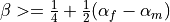.

• The method is second-order accurate provided. This is used when gamma is None.

• High frequency dissipation is maximized for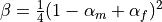. This is used when beta is None.

• The default values of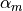,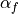(if alpha_m or alpha_f are None) are based on the user specified high-frequency dissipation parameter rho_inf.

Special settings:

•corresponds to the HHT-method.

•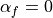corresponds to the WBZ-method.

•,produces the Newmark method.

 J. Chung, G.M.Hubert. “A Time Integration Algorithm for Structural Dynamics with Improved Numerical Dissipation: The Generalized-Method” ASME Journal of Applied Mechanics, 60, 371:375, 1993.

Kind: ‘ts.generalized_alpha’

For common configuration parameters, see Solver.

Specific configuration parameters:

Parameters
t0float (default: 0.0)

The initial time.

t1float (default: 1.0)

The final time.

dtfloat

The time step. Used if n_step is not given.

n_stepint (default: 10)

The number of time steps. Has precedence over dt.

is_linearbool (default: False)

If True, the problem is considered to be linear.

rho_inffloat (default: 0.5)

The spectral radius in the high frequency limit (user specified high-frequency dissipation) in [0, 1]: 1 = no dissipation, 0 = asymptotic annihilation.

alpha_mfloat

The parameter.

alpha_ffloat

The parameter.

betafloat

The Newmark-like parameter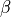.

gammafloat

The Newmark-like parameter.

create_nlst(nls, dt, alpha_m, alpha_f, gamma, beta, u0, v0, a0)[source]
name = 'ts.generalized_alpha'
class sfepy.solvers.ts_solvers.NewmarkTS(conf, nls=None, context=None, **kwargs)[source]

Solve elastodynamics problems by the Newmark method.

The method was introduced in . Common settings :

name

kind

beta

gamma

Omega_crit

trapezoidal rule:

implicit

1/4

1/2

unconditional

linear acceleration:

implicit

1/6

1/2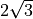Fox-Goodwin:

implicit

1/12

1/2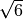central difference:

explicit

0

1/2

2

All of these methods are 2-order of accuracy.

 Newmark, N. M. (1959) A method of computation for structural dynamics. Journal of Engineering Mechanics, ASCE, 85 (EM3) 67-94.

 Arnaud Delaplace, David Ryckelynck: Solvers for Computational Mechanics

Kind: ‘ts.newmark’

For common configuration parameters, see Solver.

Specific configuration parameters:

Parameters
t0float (default: 0.0)

The initial time.

t1float (default: 1.0)

The final time.

dtfloat

The time step. Used if n_step is not given.

n_stepint (default: 10)

The number of time steps. Has precedence over dt.

is_linearbool (default: False)

If True, the problem is considered to be linear.

betafloat (default: 0.25)

The Newmark method parameter beta.

gammafloat (default: 0.5)

The Newmark method parameter gamma.

create_nlst(nls, dt, gamma, beta, u0, v0, a0)[source]
name = 'ts.newmark'
class sfepy.solvers.ts_solvers.SimpleTimeSteppingSolver(conf, nls=None, context=None, **kwargs)[source]

Implicit time stepping solver with a fixed time step.

Kind: ‘ts.simple’

For common configuration parameters, see Solver.

Specific configuration parameters:

Parameters
t0float (default: 0.0)

The initial time.

t1float (default: 1.0)

The final time.

dtfloat

The time step. Used if n_step is not given.

n_stepint (default: 10)

The number of time steps. Has precedence over dt.

quasistaticbool (default: False)

If True, assume a quasistatic time-stepping. Then the non-linear solver is invoked also for the initial time.

name = 'ts.simple'
output_step_info(ts)[source]
solve_step(ts, nls, vec, prestep_fun=None)[source]
solve_step0(nls, vec0)[source]
class sfepy.solvers.ts_solvers.StationarySolver(conf, nls=None, context=None, **kwargs)[source]

Solver for stationary problems without time stepping.

This class is provided to have a unified interface of the time stepping solvers also for stationary problems.

Kind: ‘ts.stationary’

For common configuration parameters, see Solver.

Specific configuration parameters:

name = 'ts.stationary'
class sfepy.solvers.ts_solvers.VelocityVerletTS(conf, nls=None, context=None, **kwargs)[source]

Solve elastodynamics problems by the velocity-Verlet method.

The algorithm can be found in .

 Swope, William C.; H. C. Andersen; P. H. Berens; K. R. Wilson (1 January 1982). “A computer simulation method for the calculation of equilibrium constants for the formation of physical clusters of molecules: Application to small water clusters”. The Journal of Chemical Physics. 76 (1): 648 (Appendix). doi:10.1063/1.442716

Kind: ‘ts.velocity_verlet’

For common configuration parameters, see Solver.

Specific configuration parameters:

Parameters
t0float (default: 0.0)

The initial time.

t1float (default: 1.0)

The final time.

dtfloat

The time step. Used if n_step is not given.

n_stepint (default: 10)

The number of time steps. Has precedence over dt.

is_linearbool (default: False)

If True, the problem is considered to be linear.

create_nlst(nls, dt, u0, v0, a0)[source]
name = 'ts.velocity_verlet'
sfepy.solvers.ts_solvers.adapt_time_step(ts, status, adt, context=None, verbose=False)[source]

Adapt the time step of ts according to the exit status of the nonlinear solver.

The time step dt is reduced, if the nonlinear solver did not converge. If it converged in less then a specified number of iterations for several time steps, the time step is increased. This is governed by the following parameters:

• red_factor : time step reduction factor

• red_max : maximum time step reduction factor

• inc_factor : time step increase factor

• inc_on_iter : increase time step if the nonlinear solver converged in less than this amount of iterations…

• inc_wait : …for this number of consecutive time steps

Parameters
tsVariableTimeStepper instance

The time stepper.

statusIndexedStruct instance

The nonlinear solver exit status.

adtStruct instance

The object with the adaptivity parameters of the time-stepping solver such as red_factor (see above) as attributes.

contextobject, optional

The context can be used in user-defined adaptivity functions. Not used here.

Returns
is_breakbool

If True, the adaptivity loop should stop.

sfepy.solvers.ts_solvers.gen_multi_vec_packing(size, num)[source]
sfepy.solvers.ts_solvers.get_min_dt(adt)[source]
sfepy.solvers.ts_solvers.standard_ts_call(call)[source]

Decorator handling argument preparation and timing for time-stepping solvers.